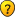## Number of possible 16x16 sudoku grids?

Everything about Sudoku that doesn't fit in one of the other sections

### Re: Number of possible 16x16 sudoku grids?

Mathimagics wrote:
To begin with we would need to identify equivalence (conjugacy) classes. The only software for this is a group-theory package like GAP. The number of VPT's (Validity Preserving Transformations) involved, is, I believe, 2 x (12 ^ 10) = 123,834,728,448 for 16x16 Sudoku, compared with just 2 x 6^8 = 3,359,232 for 9x9 Sudoku.

With a group of that size, it's highly unlikely that any desktop PC is going to be able to accomplish even this first step! Some serious architecture would need to be thrown at the problem ...

Mathimagics wrote:
coloin wrote:? isnt VPT = 24^4,24^4,24^4,24^4 * 2 = 24^16 *2 = 24233149581890213117952 = 2e22I think that the number of VPT's is actually 2 x (24 ^ 10) = 126,806,761,930,752

• band/stack permutations: 24 ^ 2
• rows/cols in band/stack: 24 ^ 8
• transposition: 2

[I see that I gave this count as 2 x 12^10 earlier on in this thread, unaccountably setting 4! = 12 (oops)Nobody picked up on this at the time ....]

Better all together, instead of just me.

Many brains think better than one

Maq777 wrote:On the wikipedia page where these topics are discussed

https://en.wikipedia.org/wiki/Mathematics_of_Sudoku

Are a table in which they give the value of for all square sudoku puzzles, and for the 16x16 case they use the value of [(4!)^10 ]× 2 × 16! for "Size of VPT group".

The only thing to do to obtain that value in sudokus nxn is to multiply the Geometric Transformations by the relabels

Size of VPT group = [Geometric Transformations] * [Relabels]

or what i called

Maximum range: [2*(n!)^((2n)+2)] * [(n^2)!]

4x4
-------------------------------------------
Geometric Transformations: 128
Relabeling :24
Maximum range :3072 (VPT)

9x9
-------------------------------------------
Geometric Transformations: 3359232
Relabeling :362880
Maximum range :1218998108160 (VPT)

16x16
------------------------------------------
Geometric Transformations: 126806761930752
Relabeling: 20922789888000
Maximum range: 2,65315E+27 (VPT)

http://forum.enjoysudoku.com/equations-that-work-for-any-sudoku-of-the-form-n-n-t38848.html

or am I wrong about something?
Maq777

Posts: 48
Joined: 30 April 2016

Previous

Return to General